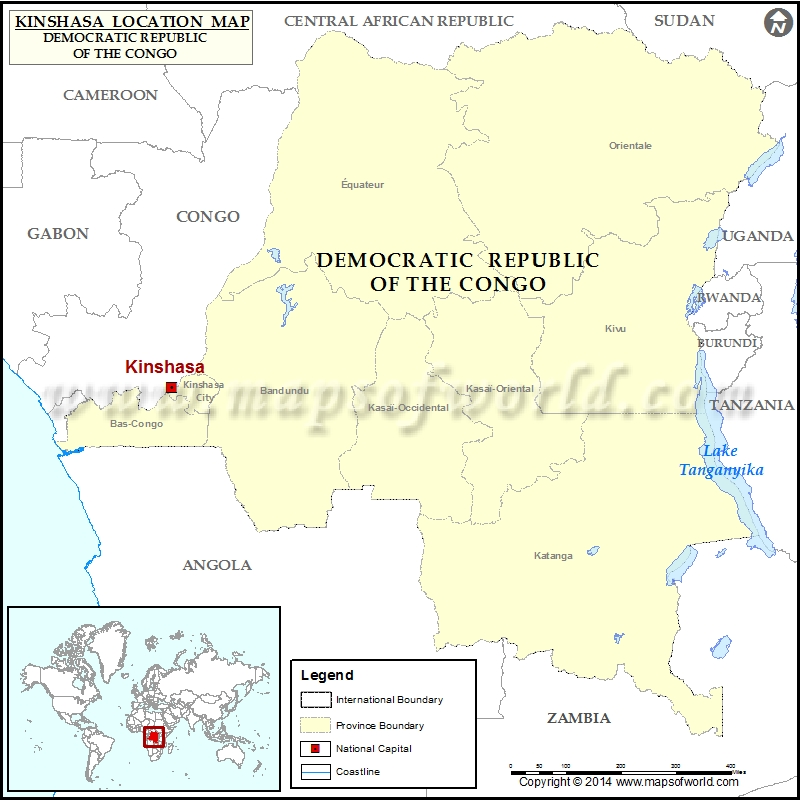﻿ Where is Kinshasa | Location of Kinshasa in Democratic Republic of the Congo Map

# Where is KinshasaDescription :  Map showing location of Kinshasa in Democratic Republic Of Congo. Disclaimer

 Close Disclaimer : All efforts have been made to make this image accurate. However Compare Infobase Limited,its directors and employees do not own any responsibility for the correctness or authenticity of the same.

Search For Latitude & Longitude Map

By Country

Or

Latitude

Longitude

Or

Area

* Put South & West values with minus ( - ) sign.

 Location Maps of Cities in Democratic Republic Of Congo
 Where is Aba Where is Aketi Where is Ango Where is Aru Where is Bafwasende Where is Bagata Where is Bakwa-Kalonji Where is Balamba Where is Bambesa Where is Banana Where is Bandundu Where is Banningville Where is Baraka Where is Basankusu Where is Basoko Where is Befale Where is Beni Where is Bikoro Where is Binga Where is Boende Where is Bokoro Where is Bokungu Where is Bolobo Where is Bolomba Where is Boma Where is Bomongo Where is Bondo Where is Bongandanga Where is Bosobolo Where is Budjala Where is Bukama Where is Bukavu Where is Bulungu Where is Bumba Where is Bunia Where is Businga Where is Buta Where is Butembo Where is Dekese Where is Demba Where is Dibaya Where is Dibaya-Lubue Where is Dilolo Where is Dilunga Where is Dimbelenge Where is Djugu Where is Dungu Where is Elizabethville Where is Feshi Where is Fizi Where is Fungurume Where is Gandajika Where is Gbadolite Where is Gemena Where is Goma Where is Gungu Where is Gwane Where is Idiofa Where is Idjwi Where is Ikela Where is Ilebo Where is Inga Where is Ingende Where is Inongo Where is Irumu Where is Isangi Where is Isiro Where is Kabalo Where is Kabambare Where is Kabinda Where is Kabongo Where is Kahemba Where is Kalemie Where is Kalima Where is Kalo Where is Kambove Where is Kamina Where is Kamituga Where is Kampene Where is Kananga Where is Kaniama Where is Kanteba Where is Kanyabayonga Where is Kapanga Where is Kasangulu Where is Kasenga Where is Kasongo Where is Kasongo-Lunda Where is Katako-Kombe Where is Katanda Where is Katwa Where is Kayna Where is Kazumba Where is Kenankuna Where is Kenge Where is Kibombo Where is Kikondja Where is Kikwit Where is Kimpese Where is Kindu Where is Kinshasa Where is Kinzau-Mvuete Where is Kipushi Where is Kiri Where is Kirumba Where is Kisangani Where is Kisantu Where is Kitona Where is Kituku Where is Kole Where is Kolwezi Where is Kongolo Where is Kungu Where is Kutu Where is Libenge Where is Likasi Where is Lisala Where is Lodja Where is Lomela Where is Lubao Where is Lubefu Where is Lubero Where is Lubudi Where is Lubumbashi Where is Lubutu Where is Luebo Where is Luiza Where is Lukolela Where is Lukula Where is Luozi Where is Lupatapata Where is Luputa Where is Lusambo Where is Lwambo Where is Mahagi Where is Malemba-Nkulu Where is Mangai Where is Mangina Where is Mankanza Where is Manono Where is Masi-Manimba Where is Masisi Where is Matadi Where is Mbandaka Where is Mbanza-Ngungu Where is Mbuji-Mayi Where is Miabi Where is Mitwaba Where is Moba Where is Mobayi-Mbongo Where is Mokambo Where is Mongbwalu Where is Monkoto Where is Muanda Where is Mulongo Where is Mushie Where is Mutshatsha Where is Mweka Where is Mwene-Ditu Where is Niangara Where is Nioki Where is Nyunzu Where is Oicha Where is Opala Where is Oshwe Where is Paulis Where is Poko Where is Popokabaka Where is Punia Where is Pweto Where is Rutshuru Where is Sakania Where is Sandoa Where is Sedzo Where is Seke-Banza Where is Shabunda Where is Shinkolobwe Where is Songololo Where is Tshela Where is Tshikapa Where is Tshilenge Where is Tshimbulu Where is Ubundu Where is Uvira Where is Vivi Where is Walikale Where is Walungu Where is Wamba Where is Watsa Where is Yahuma Where is Yakoma Where is Yangambi Where is Zaba Where is Zongo